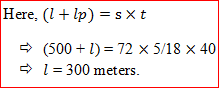New Students Offer - Use Code HELLO

# Speed, Time and Distance Questions for SBI PO: Part 4Question1: A train having length 600 meters crosses a car going in the same direction at a speed of 12 km/hr. in 45 seconds. The same train crosses another man going on bike in opposite direction to that of train in 20 seconds. Find the speed of the other man on bike in km/hr.?

Solution:
This is the case of moving object without length.
Let the speed of the train be ‘s’.
Since speed of the man in car = 12 km/hr. = 12⨯5/18 = 10/3 m/sec.

So,negative sign means both the train and the car are moving in same directionNow, let the speed of the bike be ‘s0’ m/sec.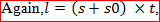positive sign is used here because both the train and the bike are going in opposite direction.Now speed of bike in km/hr. = 40/3⨯18/5 = 48km/hr.

Question2: There are two trains each having same length, they crossed a man standing on a platform in 10 second and 15 second respectively. Find the time in which the two trains will cross each other completely while:

i) Going in the same direction.
ii) Going in opposite direction.

Solution:
Let the length of each train be ‘l’ meters and their let their speeds be ‘s1’ m/sec and ‘s2’ m/sec.i) Train going in same direction.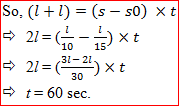i) Train going in same direction.Question3: A train running with the speed of 60 km/hr. crosses a train going with 42 km/hr. in the same direction. A person sitting in the faster train observes that it had taken 1.2 minutes to completely cross the slower train. Find the length of the slower train.

Solution:
Here we will not consider moving object with length rather we will go for moving object without length. Because the reference here is a person not the train in which he is sitting. Also the speed of that man will be considered equal to the speed of the train in which he is sitting.
So, speed of man = 60 km/hr. and time = 1.2 minutes = 12/10⨯60 = 72 seconds.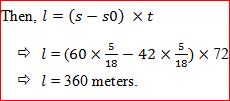Question4: A train having speed 72 km/hr. A person sitting in the train observes that the train passes a bridge completely in 25 seconds. Another man standing on the ground observes that the same train takes 40 seconds to cross the bridge completely. Find the length of the train.

Solution:
Here there are two cases:
Case 1: When the person sitting in the train crosses the bridge. (t = 25sec)
This means it is the case of moving object with no length. Let the length of platform be ‘lp’. Then length of the bridge:
Since, Distance = speed⨯ time.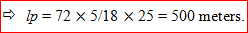Case 1: When the person standing on the ground had seen train crossing the bridge. (t = 40sec).
Let the length of train be ‘l’ meters.